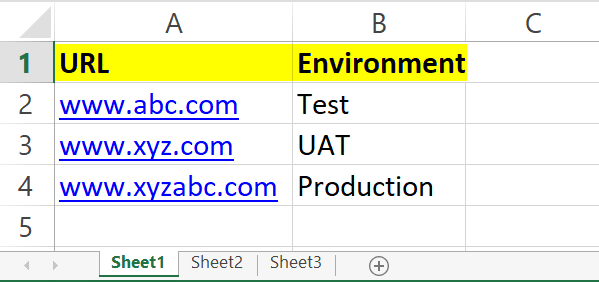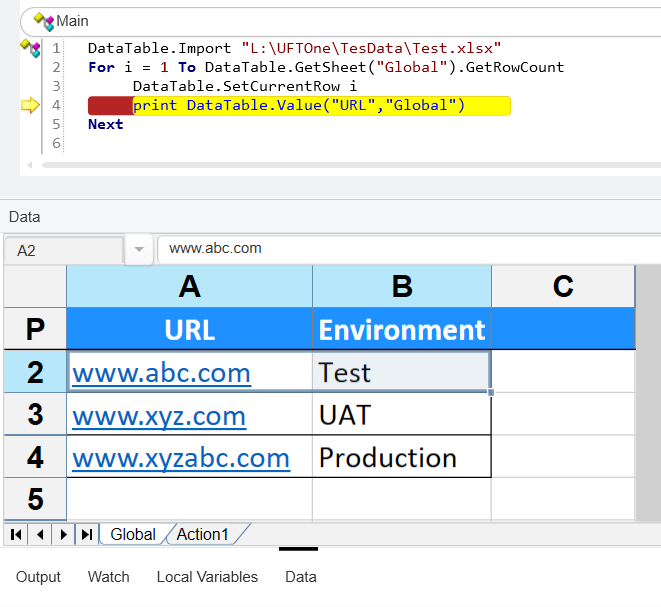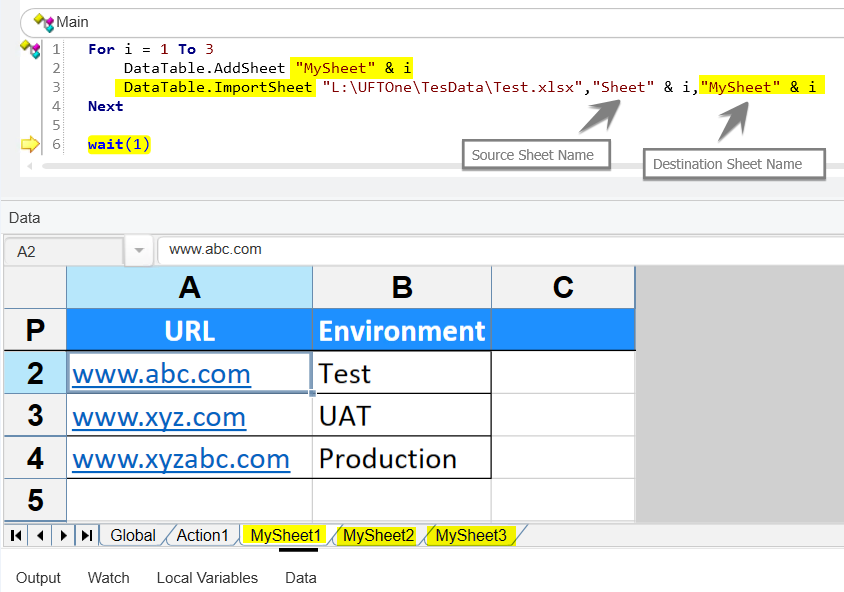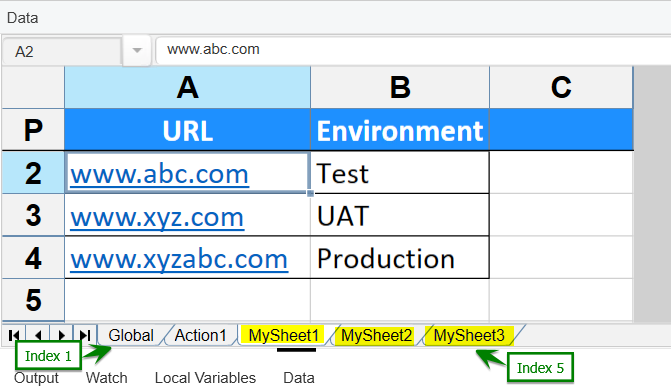# Import an Excel File into Datatable in UFT

UFT is now known as UFT One with version 15.0 onwards. In this tutorial, I will show you how to import an excel file into Datatable. I will also show you how to import only the desired excel sheet into the UFT datatable.

So, let’s start.

### DataTable.Import in UFT | How to Import an Excel file into Datatable in UFT

When we import Excel into UFT datatable the top row of the excel sheets becomes the header of the UFT Datatable. So make sure you have given valid names to the top rows as shown in the following snapshot.The Syntax to import the entire Excel file is as follows.

DataTable.Import(FileName)

So to import an excel file we can simply use the following code.

DataTable.Import “L:\UFTOne\TesData\Test.xlsx”

It will import the first two sheets of the excel UFT Datatable. The first sheet will be imported to Global Sheet and the second excel sheet will be imported to the local sheet i.e Action1.Note: DataTable.Import method can only import up to first two sheets of the excel file in UFT DataTable.If you want to more than two excel sheets to UFT DataTable , you can use DataTable.ImportSheet method

### DataTable.ImportSheet in UFT | Import a specific Excel sheet into UFT DataTable

DataTable.ImportSheet method is quite useful if you want to import any specific excel sheet to UFT DataTable.

The Syntax to import the desired Excel Sheet is as follows.

DataTable.ImportSheet FileName, vtSourceSheet, vtDestinationSheet

So to import the desired excel sheet we can simply use the following code.

DataTable.ImportSheet “L:\UFTOne\TesData\Test.xlsx” , “Sheet2” , “Action1”

Sheet2
from the Excel file will be imported into UFT local sheet i.e Action1.

### How to Import Multiple Excel Sheets into UFT DataTable

In the above example, we have seen how to import the desired excel sheet into UFT datatable using DataTable.ImportSheet. We can use the ImportSheet method to import multiple excel sheets into UFT datatable using a for a loop.

In this example, we will import three excel sheets into UFT Datatable. For your better understanding, I will add a new local sheet in UFT datatable and import the required excel sheet into it and repeat the same for two more sheets.

I have taken a for loop and using the loop counter dynamically adding destination local sheets into UFT Datatable with names MySheet1, MySheet1, and MySheet3 and importing excel sheets into these local sheets.### DataTable.DeleteSheet in UFT | How to Delete Sheet in UFT Datatable

It is a part of best practices to delete runtime added local sheets from UFT Datatabe after their purpose has been fulfilled.

The Syntax to delete sheet is as follows.

DataTable.DeleteSheet SheetID

You can delete a sheet either by its SheetID/SheetName or its Index. So to import the desired excel sheet we can simply use the following code.

Consider the following snapshot.We can delete MySheet1 by using its index the following way

DataTable.DeleteSheet (3)

We can delete MySheet1 by using its ID/Name in the following way

DataTable.DeleteSheet (“MySheet1”)